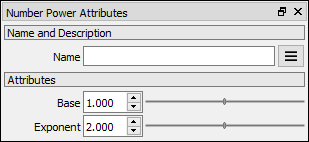# Number Power node

Left Toolbar >Math Nodes >Number Power

The Number Power node allows you to execute a exponentiation operation with two Number values.

## Procedure

### To execute a power operation on two Node Values in the node graph:

1. On the left toolbar choose Math Nodes> Number Power2. Drag the Number Power icon into the Node Graph.3. Connect the Output: result.

## Inputs

base A NUMBER type of value.

exponent A NUMBER type of value.

## Outputs

result The number value.

## Attributes PanelBase The first term in the exponentiation operation. This attribute is exposed as input by default.

Exponent The second term in the exponentiation operation. This attribute is exposed as input by default.

Math Nodes

Main Page